# Shuffle algebra

(diff) ← Older revision | Latest revision (diff) | Newer revision → (diff)

Letbe a set (alphabet) and consider the free associative algebra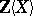onover the integers, provided with the Hopf algebra structure given by,,. As an Abelian group,is free and graded. Its graded dual is again a Hopf algebra, sometimes called the shuffle-cut Hopf algebra or merge-cut Hopf algebra. Its underlying algebra is the shuffle algebra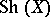. As an Abelian group,has as basis the elements of the free monoid (see Free semi-group)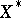of all words in the alphabet. The product of two such words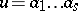,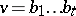is the sum of all words of length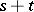that are permutations of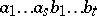such that bothandappear in their original order. E.g.,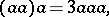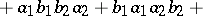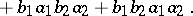This is the shuffle product. It derives its name from the familiar rifle shuffle of decks of playing cards.

As an algebra over,is a free commutative algebra with as free commutative generators the Lyndon words in, see Lyndon word. I.e.,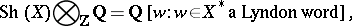[a1]. It is not true thatis free over.

How to Cite This Entry:
Shuffle algebra. Encyclopedia of Mathematics. URL: http://encyclopediaofmath.org/index.php?title=Shuffle_algebra&oldid=11572
This article was adapted from an original article by M. Hazewinkel (originator), which appeared in Encyclopedia of Mathematics - ISBN 1402006098. See original article